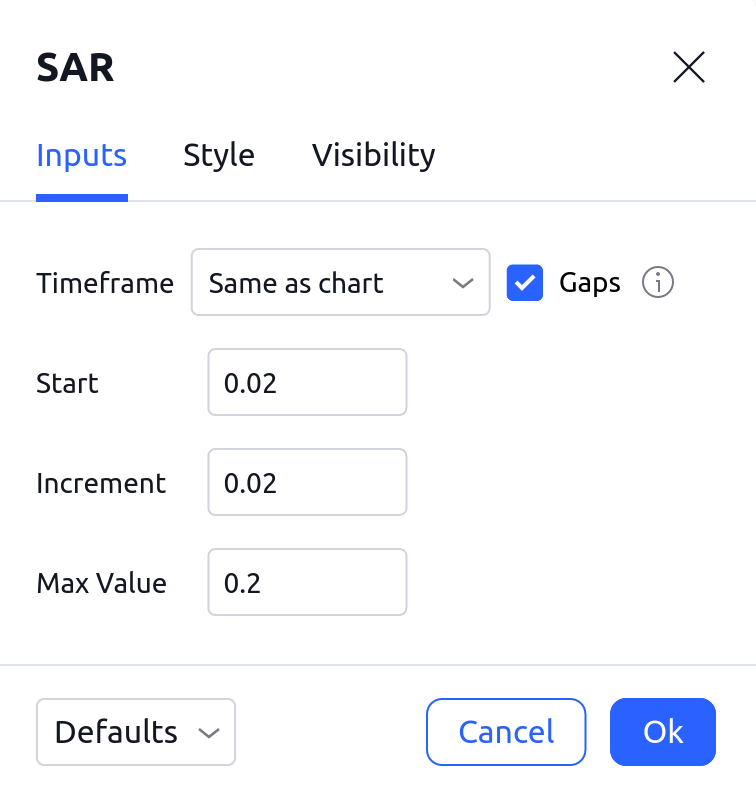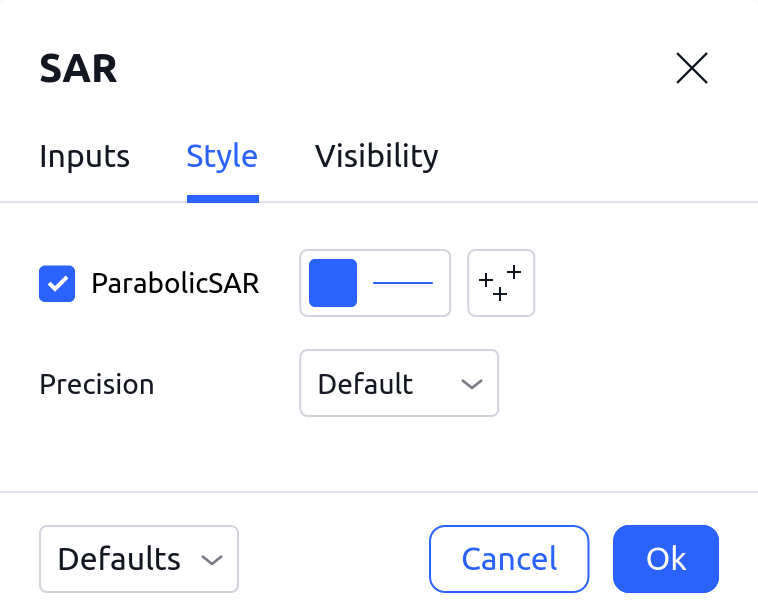Parabolic SAR (SAR)

Definition

Parabolic SAR (SAR) is a time and price technical analysis tool primarily used to identify points of potential stops and reverses. In fact, the SAR in Parabolic SAR stands for "Stop and Reverse". The indicator's calculations create a parabola which is located below price during a Bullish Trend and above Price during a Bearish Trend.

History

J. Welles Wilder created the Parabolic SAR (SAR) and featured it in his book New Concepts in Technical Trading Systems. The book was published in 1978 and also featured several of his now classic indicators such as; The Relative Strength Index, Average True Range and the Directional Movement Index. Much like the indicators mentioned, the Parabolic SAR indicator is still widely used and has great importance in the world of technical analysis.

Calculation

We have adopted the original algorithm for the Parabolic SAR (which stands for stop and reverse) described in the book New Concepts in Technical Trading Systems written by the creator of SAR, J. Welles Wilder Jr.

To first understand Parabolic SAR, it’s important to know how it looks on the chart. SAR is most commonly displayed as a dotted line that is charted alongside an asset’s price. Generally, when an asset is rising, SAR is displayed below the price and when an asset is falling, SAR is displayed above the price. A buy or reversal is signaled when the price crosses above or below Parabolic SAR.

The steps look like this:

1. For the first day of entry or reversal, the SAR is the previous EP (Extreme Point) - the highest high of the uptrend or the lowest low of the downtrend.

A. If entered Long (uptrend), the EP is the lowest price reached while in the previous Short trade.

B. If entered Short (downtrend), the EP is the highest price reached while in the previous Long trade.

2. For the second day and thereafter, the SAR is calculated in a specific manner depending on if the asset is in an uptrend or downtrend. If the asset is in an uptrend, the calculation looks like this:

A. Find the difference between the highest price made while in the trade (EP) and the SAR for that day. Multiply the difference by the AF (Acceleration Factor - determines the sensitivity of the SAR) and add the result to the current SAR to obtain the SAR for the next day.

B. Use the Start Value (see image below, 0.02 by default) for the first AF and increase its Increment (0.02 by default) on each day a new high for the trade is made. If a new high is not made, continue to use the AF as it was last increased. Do not increase the AF above the Max Value (0.2 by default).If the asset is in a downtrend, the calculation looks like this:

A. Find the difference between the lowest price made while in the trade (EP) and the SAR on its current day. Multiply the difference by the AF and add the result to the current SAR to obtain the SAR for tomorrow.

B. Use Start value (0.02 by default) for the first AF and increase its Increment (0.02 by default) on each day a new low for the trade is made. If a new low is not made, continue to use the AF as it was last increased. Do not increase the AF above the Max Value (0.2 by default).

3. As a final step, it’s important to remember to never move SAR into the previous day’s range or any day before that.

A. If in an uptrend, never move the current SAR above the previous day’s low or the day before that. If SAR is calculated to be above those values, then use the lower low between the previous day and the day before that as the new SAR. Make the next day’s calculations based on this.

B. If in a downtrend, never move the current SAR below the previous day’s high or the day before that. If the SAR is calculated to be below those values, then use the higher high the previous day and the day before that as the new SAR. Make the next day’s calculations based upon this SAR.

Let’s sum it up!

First, for every new bar, we calculate the current SAR based on previous values (see step 2 from the rules above).

Previous SAR + Previous AF * (Previous EP - Previous SAR) = Current SAR

Second, we check if the calculated value is penetrated by the price of a current bar. We use < and > inequality operators to compare values. If it is, a reversal is made and the previous EP as a new SAR value (see paragraph 1 in the rules above).

If there is no reversal, we check if the calculated SAR value is above the two previous low values in case of uptrend or below the two previous high values in case of downtrend (see paragraph 3 in the rules above). If it is, we update the SAR value according to the rules.

On every new bar we update the AF value if EP value gets updated (see paragraph 2 in the rules above).

How SAR is calculated since the beginning of its history

In the book that introduced SAR, there are no clear instructions on how to calculate SAR for the beginning of a barset. Therefore, we assume that the SAR value on the first bar cannot be calculated because there are no previous values. On a second bar, we define if a trend is up or down, comparing the close values of the first and second bars. Then, we use the rule from paragraph 1 in the rules above: we use high of the first bar as a SAR on a second bar if a trend is down, and low of the first value if a trend is up.

What to look for

Stops and Reversals

The Parabolic SAR (SAR) indicator has a very simple premise. When price in an uptrend breaks below the parabola (which will be below the trend), this signals a potential reversal of price. When price breaks above the parabola (above the trend) during a downtrend, this can also signal a price reversal.
In a practical sense, many traders use these breakthroughs as a point to place stop orders or even as points to enter the market at the beginning of a new trend.

Summary

Parabolic SAR is a nice, compact indicator that can provide some good information. It is not typically recommended, however to use it as a stand-alone to generate trading signals. Since it is time and price based it is not adept at measuring the actual strength of a trend, merely its direction and duration. It is a good idea to use it in conjunction with an indicator that specializes in measuring trend strength. A good choice would be Wilder's own Directional Movement.

InputsIndicator Timeframe

Specifies the timeframe that the indicator is calculated on. This option allows calculating SAR based on a data from another timeframe, e.g. having SAR calculated on 1H chart be displayed on a 5m chart.

Start

The starting value for the Acceleration Factor (.02 is the Default).

Increment

The increment in which the Acceleration Factor will move (.02 is Default).

Max Value

The maximum value of the Acceleration Factor (.20 is the Default)

StyleParabolic SAR

Can toggle the visibility of the Parabolic SAR as well as the visibility of a price line showing the actual current value of the Parabolic SAR. Can also select the Parabolic SAR's color, cross thickness and visual style (Cross is the Default).